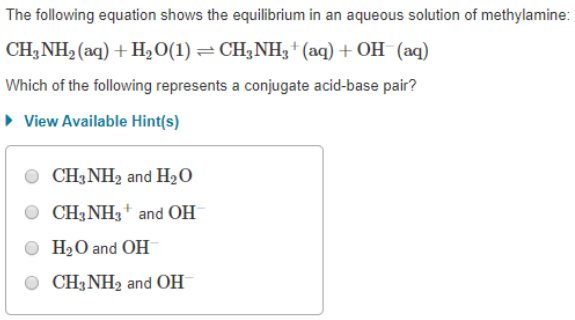# The following equation shows the equilibrium in an aqueous solution of methylamine: CH3NH2(aq) + H2O(1) ⇌ CH3NH3^+(aq) + OH^-(aq) Which of the following represents a conjugate acid-base pair? a) CH3NH2 and H2O b) CH3NH3^+ and OH^- c) H2O and OH^- d) CH3NH2 and OH^-